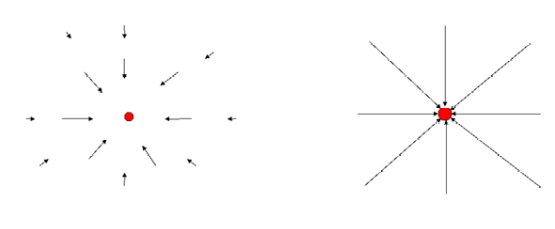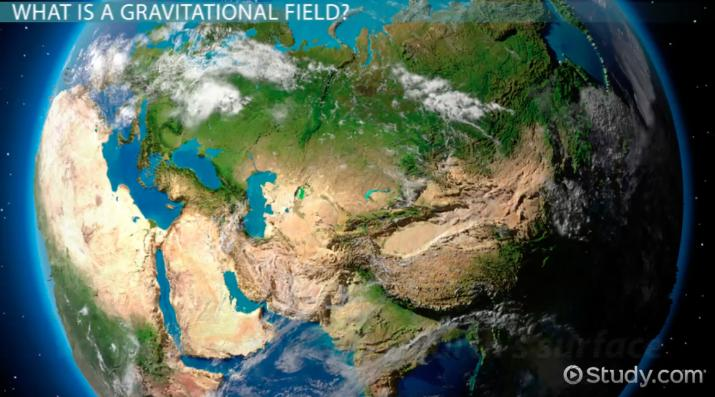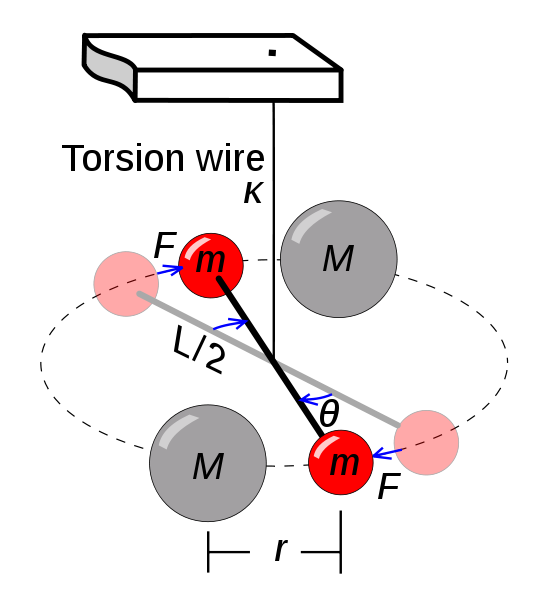Science Courses / Course / Chapter

# Gravitational Field: Definition & Formula

Lesson Transcript
Instructor: Thomas Zesiger

Thomas has taught electronics and communications engineering, math, and physics and has a master's degree in electrical engineering.

In space, there is a force field that exists around every mass or group of masses that is known as the gravitational field. Understand the definition of a gravitational field, and the formula used to calculate gravitational force.

## What Is a Gravitational Field?

A gravitational field is the force field that exists in the space around every mass or group of masses. This field extends out in all directions, but the magnitude of the gravitational force decreases as the distance from the object increases. It is measured in units of force per mass, usually newtons per kilogram (N/kg). A gravitational field is a type of force field and is analogous to electric and magnetic fields for electrically charged particles and magnets, respectively.

There are two ways of showing the gravitational field around an object: with arrows and with field lines. Both of these are shown in the picture below. Arrows show the magnitude and direction of the force at different points in space. The longer the arrow, the greater the magnitude. Field lines show the direction the force would act on an object placed at that point in space. The magnitude of the field is represented by the spacing of the lines. The closer the lines are to each other, the higher the magnitude.The gravitational field varies slightly at the earth's surface. For example, the field is slightly stronger than average over subterranean lead deposits. Large caverns that may be filled with natural gas have a slightly weaker gravitational field. Geologists and prospectors of oil and minerals make precise measurements of the earth's gravitational field to predict what may be beneath the surface.An error occurred trying to load this video.

Try refreshing the page, or contact customer support.

Coming up next: Magnetic Poles: Definition & Shifts

### You're on a roll. Keep up the good work!

Replay
Your next lesson will play in 10 seconds
• 0:00 What is a Gravitational Field?
• 1:20 Formula
• 4:55 Lesson Summary
Save Timeline
Autoplay
Autoplay
Speed Speed

## Formula

The earth and moon exert a force, or pull, on each other even though they are not in contact. In other words, the two bodies interact with one another's gravitational field. Another example is the interaction of the earth and a satellite in orbit around it.

From these examples, Newton developed the law of universal gravitation. The law of universal gravitation says that every object exerts a gravitational pull on every other object. The force is proportional to the masses of both objects and inversely proportional to the square of the distance between them (or the distance between their centers of mass if they are spherical objects). Using variables, we write F is proportional to mM/d^2, where F is the force, m is the mass of the smaller object, M is the mass of the larger object, and d is the distance between the two objects.

In 1798, English physicist Henry Cavendish performed precise measurements of the actual gravitational forces acting between masses using a torsion balance. The outcome of his experiment resulted in the constant of proportionality in the law of universal gravitation called the universal gravitational constant. Inserting this into the proportionality results in the equation F = G(mM/d^2). The value for G is 6.67 x 10^-11 newton-meters squared per square kilogram (N-m^2/kg^2).

The following is a diagram of the torsion balance set up used by Cavendish to determine the universal gravitational constant.To unlock this lesson you must be a Study.com Member.

### Register to view this lesson

Are you a student or a teacher?

Back

### Resources created by teachers for teachers

Over 30,000 video lessons & teaching resources‐all in one place.Video lessonsQuizzes & WorksheetsClassroom IntegrationLesson Plans

I would definitely recommend Study.com to my colleagues. It’s like a teacher waved a magic wand and did the work for me. I feel like it’s a lifeline.

Jennifer B.
TeacherCreate an account to start this course today
Used by over 30 million students worldwide